# Solving TwoStep and Solving TwoStep 2 3 MultiStep

• Slides: 45Solving Two-Step and Solving Two-Step 2 -3 Multi-Step Equations Warm Up Lesson Presentation Lesson Quiz Holt Mc. Dougal Algebra 1Solving Two-Step and 2 -3 Multi-Step Equations Warm Up Evaluate each expression. 1. 9 – 3(– 2) 15 2. 3(– 5 + 7) 6 3. – 4 4. 26 – 4(7 – 5) 18 Simplify each expression. 5. 10 c + c 11 c 6. 8. 2 b + 3. 8 b – 12 b 0 7. 5 m + 2(2 m – 7) 9 m – 14 8. 6 x – (2 x + 5) 4 x – 5 Holt Mc. Dougal Algebra 1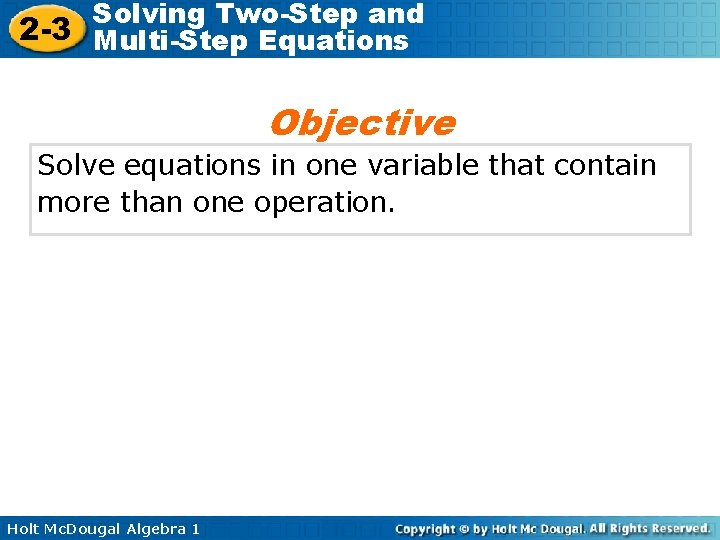Solving Two-Step and 2 -3 Multi-Step Equations Objective Solve equations in one variable that contain more than one operation. Holt Mc. Dougal Algebra 1Solving Two-Step and 2 -3 Multi-Step Equations Notice Alex belongs that this to equation a music club. contains In this multiplication club, students can buy and addition. a student Equations discount thatcard contain for \$19. 95. more than Thisone card allows operation require them to more buythan CDs one for \$3. 95 step toeach. solve. After one year, Identify the Alex operations has spent in \$63. 40. the equation and the order in which they are applied to the variable. To find the number of CDs c that Alex bought, you Then use inverse operations and work backward to can solve an equation. undo them one at a time. Cost per CD Total cost Cost of discount card Holt Mc. Dougal Algebra 1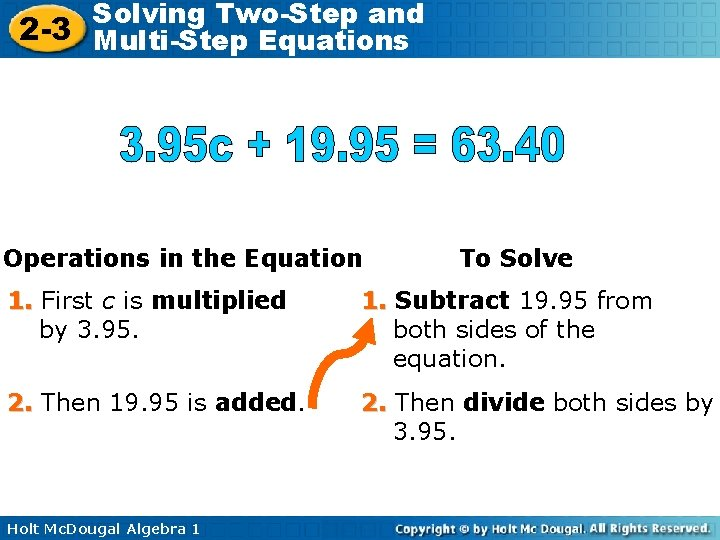Solving Two-Step and 2 -3 Multi-Step Equations Operations in the Equation To Solve 1. First c is multiplied by 3. 95. 1. Subtract 19. 95 from both sides of the equation. 2. Then 19. 95 is added. 2. Then divide both sides by 3. 95. Holt Mc. Dougal Algebra 1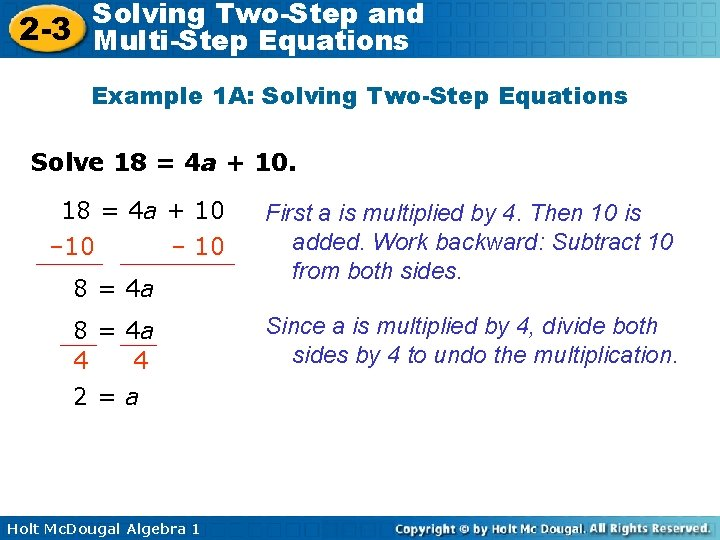Solving Two-Step and 2 -3 Multi-Step Equations Example 1 A: Solving Two-Step Equations Solve 18 = 4 a + 10 – 10 8 = 4 a 4 4 2=a Holt Mc. Dougal Algebra 1 First a is multiplied by 4. Then 10 is added. Work backward: Subtract 10 from both sides. Since a is multiplied by 4, divide both sides by 4 to undo the multiplication.Solving Two-Step and 2 -3 Multi-Step Equations Example 1 B: Solving Two-Step Equations Solve 5 t – 2 = – 32 +2 +2 5 t = – 30 5 5 t = – 6 Holt Mc. Dougal Algebra 1 First t is multiplied by 5. Then 2 is subtracted. Work backward: Add 2 to both sides. Since t is multiplied by 5, divide both sides by 5 to undo the multiplication.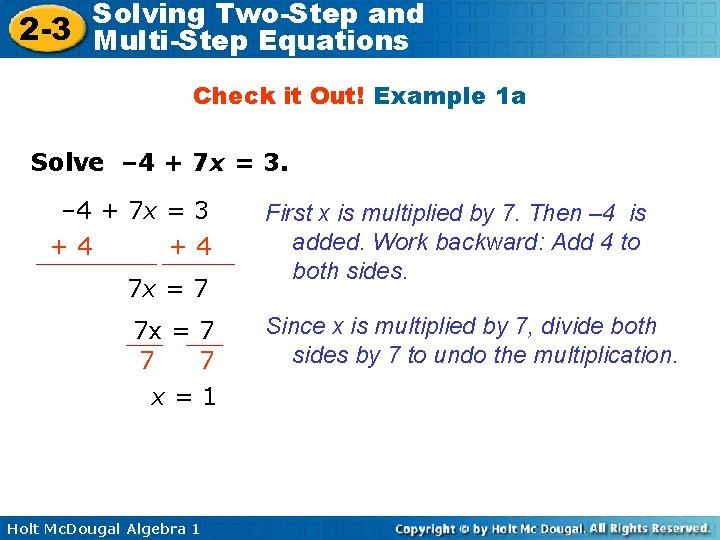Solving Two-Step and 2 -3 Multi-Step Equations Check it Out! Example 1 a Solve – 4 + 7 x = 3 +4 +4 7 x = 7 7 7 x=1 Holt Mc. Dougal Algebra 1 First x is multiplied by 7. Then – 4 is added. Work backward: Add 4 to both sides. Since x is multiplied by 7, divide both sides by 7 to undo the multiplication.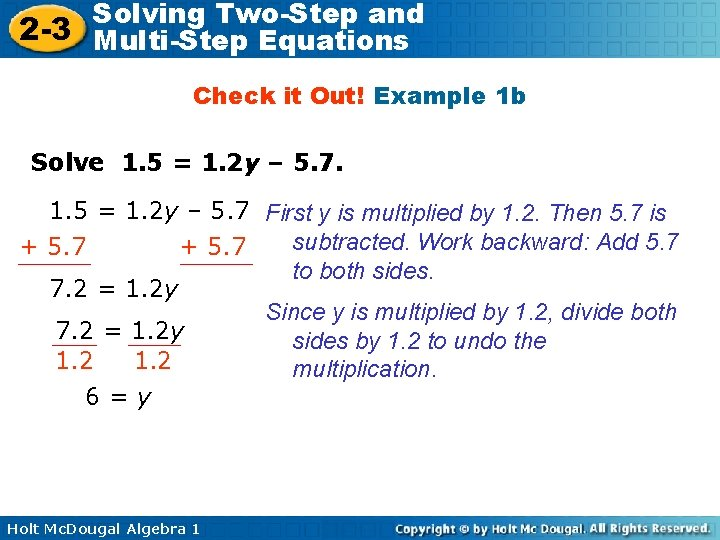Solving Two-Step and 2 -3 Multi-Step Equations Check it Out! Example 1 b Solve 1. 5 = 1. 2 y – 5. 7 First y is multiplied by 1. 2. Then 5. 7 is subtracted. Work backward: Add 5. 7 + 5. 7 to both sides. 7. 2 = 1. 2 y Since y is multiplied by 1. 2, divide both 7. 2 = 1. 2 y sides by 1. 2 to undo the 1. 2 multiplication. 6=y Holt Mc. Dougal Algebra 1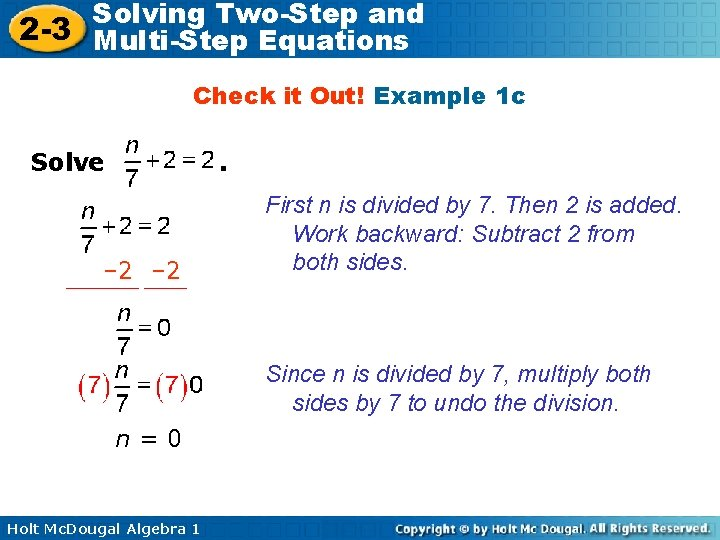Solving Two-Step and 2 -3 Multi-Step Equations Check it Out! Example 1 c Solve . – 2 First n is divided by 7. Then 2 is added. Work backward: Subtract 2 from both sides. Since n is divided by 7, multiply both sides by 7 to undo the division. n=0 Holt Mc. Dougal Algebra 1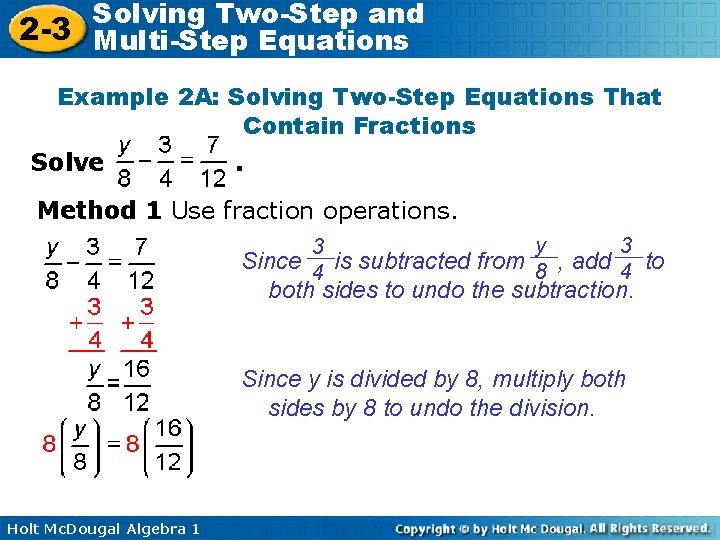Solving Two-Step and 2 -3 Multi-Step Equations Example 2 A: Solving Two-Step Equations That Contain Fractions Solve . Method 1 Use fraction operations. y 3 3 Since 4 is subtracted from 8 , add 4 to both sides to undo the subtraction. Since y is divided by 8, multiply both sides by 8 to undo the division. Holt Mc. Dougal Algebra 1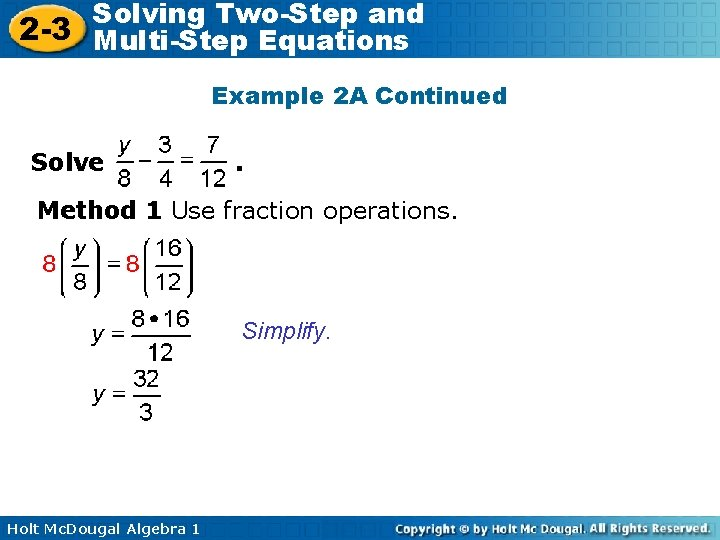Solving Two-Step and 2 -3 Multi-Step Equations Example 2 A Continued Solve . Method 1 Use fraction operations. Simplify. Holt Mc. Dougal Algebra 1Solving Two-Step and 2 -3 Multi-Step Equations Example 2 A Continued Solve . Method 2 Multiply by the LCD to clear the fractions. Multiply both sides by 24, the LCD of the fractions. Distribute 24 on the left side. 3 y – 18 = 14 +18 3 y = 32 Holt Mc. Dougal Algebra 1 Simplify. Since 18 is subtracted from 3 y, add 18 to both sides to undo the subtraction.Solving Two-Step and 2 -3 Multi-Step Equations Example 2 A Continued Solve . Method 2 Multiply by the LCD to clear the fractions. Since y is multiplied by 3, divide 3 y = 32 both sides by 3 to undo the 3 3 multiplication. Holt Mc. Dougal Algebra 1Solving Two-Step and 2 -3 Multi-Step Equations Example 2 B: Solving Two-Step Equations That Contain Fractions Solve . Method 1 Use fraction operations. 3 Since 3 is added to 2 r, subtract 4 4 3 from both sides to undo the addition. 2 3 The reciprocal of 3 is 2. Since r is 2 multiplied by , multiply both sides 3 3 by Holt Mc. Dougal Algebra 1 2 .Solving Two-Step and 2 -3 Multi-Step Equations Example 2 B Continued Solve . Method 1 Use fraction operations. Holt Mc. Dougal Algebra 1Solving Two-Step and 2 -3 Multi-Step Equations Example 2 B Continued Solve . Method 2 Multiply by the LCD to clear the fractions. Multiply both sides by 12, the LCD of the fractions. Distribute 12 on the left side. 8 r + 9 = 7 – 9 8 r = – 2 Holt Mc. Dougal Algebra 1 Simplify. Since 9 is added to 8 r, subtract 9 from both sides to undo the addition.Solving Two-Step and 2 -3 Multi-Step Equations Example 2 B Continued Solve . Method 2 Multiply by the LCD to clear the fractions. 8 r = – 2 8 8 Holt Mc. Dougal Algebra 1 Since r is multiplied by 8, divide both sides by 8 to undo the multiplication.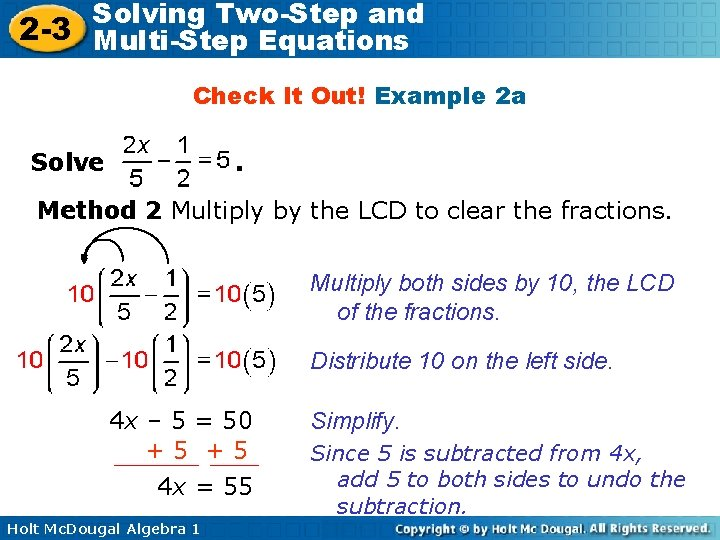Solving Two-Step and 2 -3 Multi-Step Equations Check It Out! Example 2 a Solve . Method 2 Multiply by the LCD to clear the fractions. Multiply both sides by 10, the LCD of the fractions. Distribute 10 on the left side. 4 x – 5 = 50 +5 +5 4 x = 55 Holt Mc. Dougal Algebra 1 Simplify. Since 5 is subtracted from 4 x, add 5 to both sides to undo the subtraction.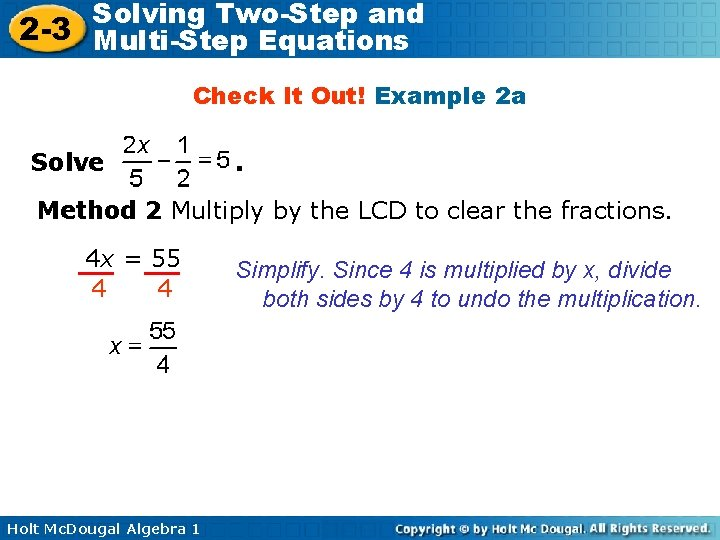Solving Two-Step and 2 -3 Multi-Step Equations Check It Out! Example 2 a Solve . Method 2 Multiply by the LCD to clear the fractions. 4 x = 55 4 4 Holt Mc. Dougal Algebra 1 Simplify. Since 4 is multiplied by x, divide both sides by 4 to undo the multiplication.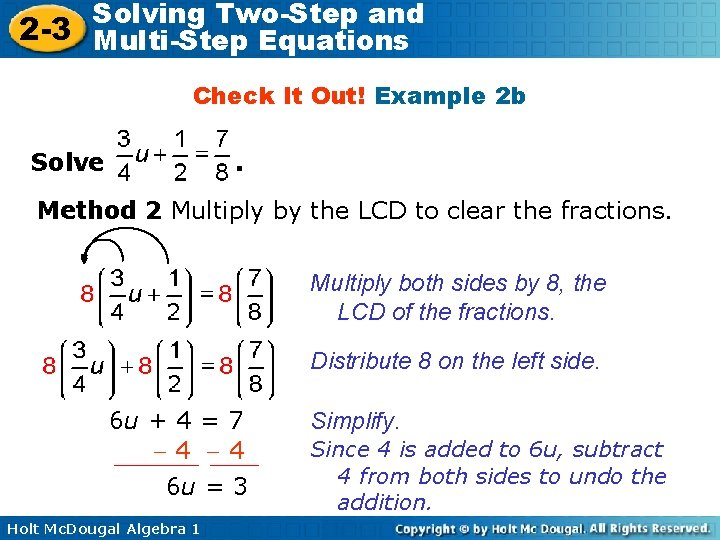Solving Two-Step and 2 -3 Multi-Step Equations Check It Out! Example 2 b Solve . Method 2 Multiply by the LCD to clear the fractions. Multiply both sides by 8, the LCD of the fractions. Distribute 8 on the left side. 6 u + 4 = 7 4 4 6 u = 3 Holt Mc. Dougal Algebra 1 Simplify. Since 4 is added to 6 u, subtract 4 from both sides to undo the addition.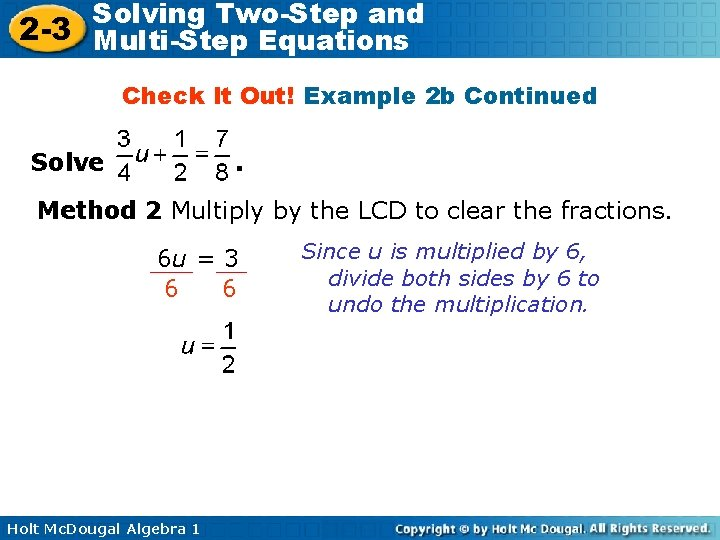Solving Two-Step and 2 -3 Multi-Step Equations Check It Out! Example 2 b Continued Solve . Method 2 Multiply by the LCD to clear the fractions. 6 u = 3 6 6 Holt Mc. Dougal Algebra 1 Since u is multiplied by 6, divide both sides by 6 to undo the multiplication.Solving Two-Step and 2 -3 Multi-Step Equations Check It Out! Example 2 c Solve . Method 1 Use fraction operations. n 1 1 Since 3 is subtracted from 5 , add 3 to both sides to undo the subtraction. Simplify. Holt Mc. Dougal Algebra 1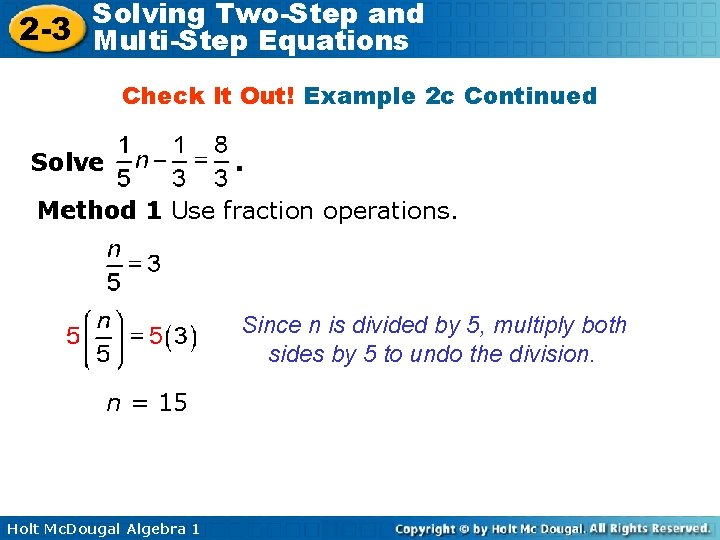Solving Two-Step and 2 -3 Multi-Step Equations Check It Out! Example 2 c Continued Solve . Method 1 Use fraction operations. Since n is divided by 5, multiply both sides by 5 to undo the division. n = 15 Holt Mc. Dougal Algebra 1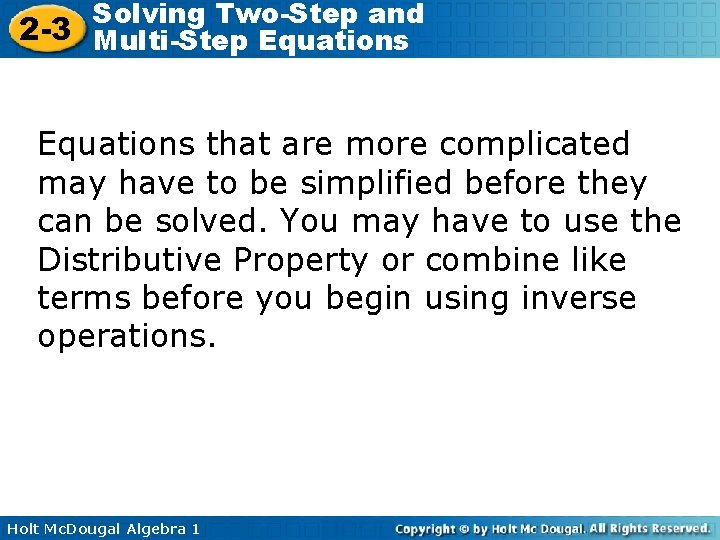Solving Two-Step and 2 -3 Multi-Step Equations that are more complicated may have to be simplified before they can be solved. You may have to use the Distributive Property or combine like terms before you begin using inverse operations. Holt Mc. Dougal Algebra 1Solving Two-Step and 2 -3 Multi-Step Equations Example 3 A: Simplifying Before Solving Equations Solve 8 x – 21 + 5 x = – 15. 8 x – 21 – 5 x = – 15 8 x – 5 x – 21 = – 15 Use the Commutative Property of Addition. 3 x – 21 = – 15 Combine like terms. + 21 +21 Since 21 is subtracted from 3 x, add 21 to both sides to undo the subtraction. 3 x = 6 Since x is multiplied by 3, divide both sides by 3 to undo the multiplication. x=2 Holt Mc. Dougal Algebra 1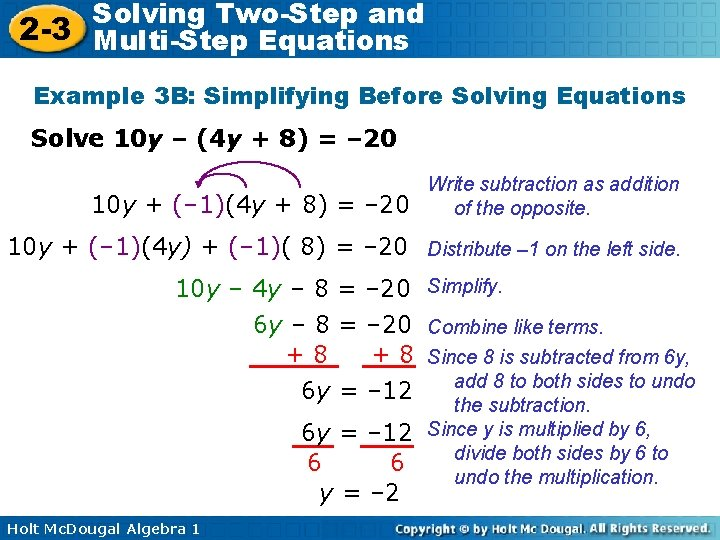Solving Two-Step and 2 -3 Multi-Step Equations Example 3 B: Simplifying Before Solving Equations Solve 10 y – (4 y + 8) = – 20 10 y + (– 1)(4 y + 8) = – 20 Write subtraction as addition of the opposite. 10 y + (– 1)(4 y) + (– 1)( 8) = – 20 Distribute – 1 on the left side. 10 y – 4 y – 8 = – 20 Simplify. 6 y – 8 = – 20 Combine like terms. +8 + 8 Since 8 is subtracted from 6 y, add 8 to both sides to undo 6 y = – 12 6 6 y = – 2 Holt Mc. Dougal Algebra 1 the subtraction. Since y is multiplied by 6, divide both sides by 6 to undo the multiplication.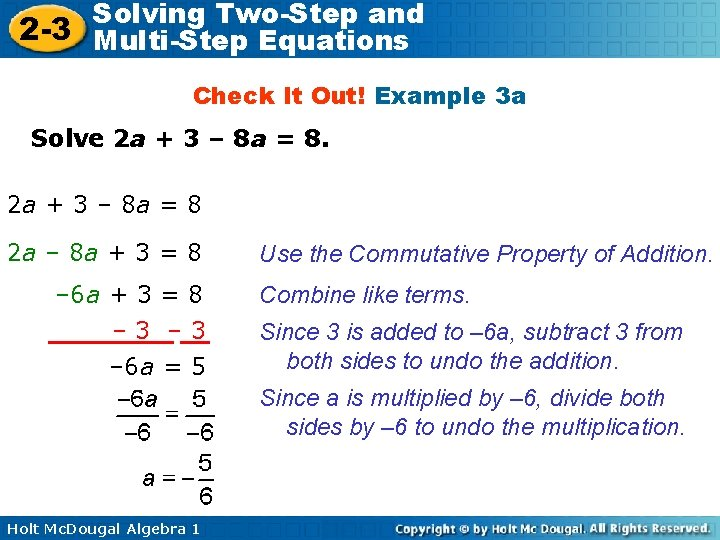Solving Two-Step and 2 -3 Multi-Step Equations Check It Out! Example 3 a Solve 2 a + 3 – 8 a = 8 2 a – 8 a + 3 = 8 – 6 a + 3 = 8 – 3 – 6 a = 5 Use the Commutative Property of Addition. Combine like terms. Since 3 is added to – 6 a, subtract 3 from both sides to undo the addition. Since a is multiplied by – 6, divide both sides by – 6 to undo the multiplication. Holt Mc. Dougal Algebra 1Solving Two-Step and 2 -3 Multi-Step Equations Check It Out! Example 3 b Solve – 2(3 – d) = 4 (– 2)(3) + (– 2)(–d) = 4 – 6 + 2 d = 4 +6 +6 2 d = 10 2 2 d=5 Holt Mc. Dougal Algebra 1 Distribute – 2 on the left side. Simplify. Add 6 to both sides. Since d is multiplied by 2, divide both sides by 2 to undo the multiplication.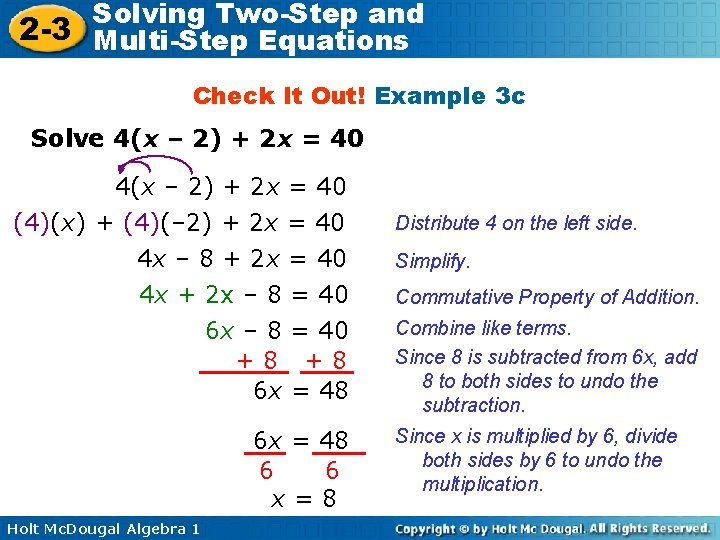Solving Two-Step and 2 -3 Multi-Step Equations Check It Out! Example 3 c Solve 4(x – 2) + 2 x = 40 (4)(x) + (4)(– 2) + 2 x = 40 4 x – 8 + 2 x = 40 4 x + 2 x – 8 6 x – 8 +8 6 x = 40 +8 = 48 6 x = 48 6 6 x=8 Holt Mc. Dougal Algebra 1 Distribute 4 on the left side. Simplify. Commutative Property of Addition. Combine like terms. Since 8 is subtracted from 6 x, add 8 to both sides to undo the subtraction. Since x is multiplied by 6, divide both sides by 6 to undo the multiplication.Solving Two-Step and 2 -3 Multi-Step Equations Example 4: Application Jan joined the dining club at the local café for a fee of \$29. 95. Being a member entitles her to save \$2. 50 every time she buys lunch. So far, Jan calculates that she has saved a total of \$12. 55 by joining the club. Write and solve an equation to find how many time Jan has eaten lunch at the café. Holt Mc. Dougal Algebra 1Solving Two-Step and 2 -3 Multi-Step Equations Example 4: Application Continued 1 Understand the Problem The answer will be the number of times Jan has eaten lunch at the café. List the important information: • Jan paid a \$29. 95 dining club fee. • Jan saves \$2. 50 on every lunch meal. • After one year, Jan has saved \$12. 55. Holt Mc. Dougal Algebra 1Solving Two-Step and 2 -3 Multi-Step Equations Example 4: Application Continued 2 Make a Plan Let m represent the number of meals that Jan has paid for at the café. That means that Jan has saved \$2. 50 m. However, Jan must also add the amount she spent to join the dining club. amount total saved dining club = on each – fee amount saved meal 12. 55 Holt Mc. Dougal Algebra 1 = 2. 50 m – 29. 95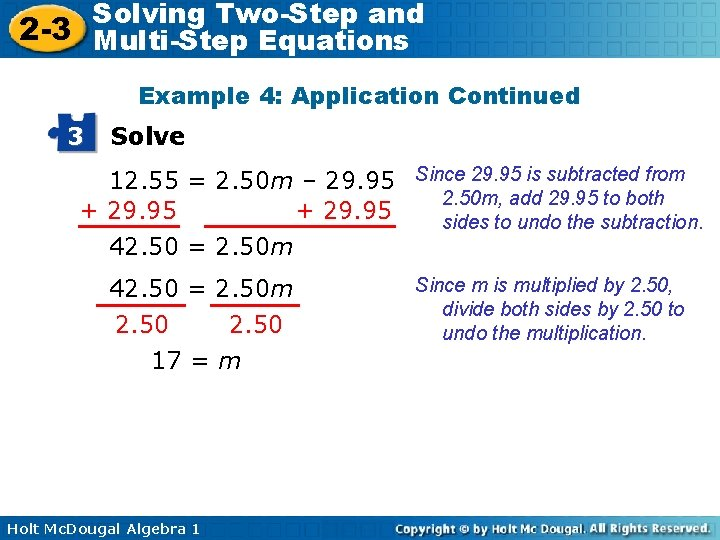Solving Two-Step and 2 -3 Multi-Step Equations Example 4: Application Continued 3 Solve 12. 55 = 2. 50 m – 29. 95 Since 29. 95 is subtracted from 2. 50 m, add 29. 95 to both + 29. 95 sides to undo the subtraction. 42. 50 = 2. 50 m 2. 50 17 = m Holt Mc. Dougal Algebra 1 Since m is multiplied by 2. 50, divide both sides by 2. 50 to undo the multiplication.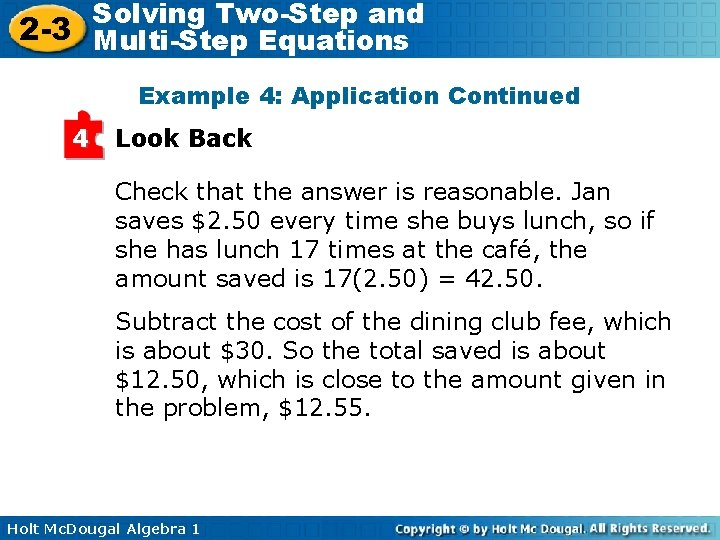Solving Two-Step and 2 -3 Multi-Step Equations Example 4: Application Continued 4 Look Back Check that the answer is reasonable. Jan saves \$2. 50 every time she buys lunch, so if she has lunch 17 times at the café, the amount saved is 17(2. 50) = 42. 50. Subtract the cost of the dining club fee, which is about \$30. So the total saved is about \$12. 50, which is close to the amount given in the problem, \$12. 55. Holt Mc. Dougal Algebra 1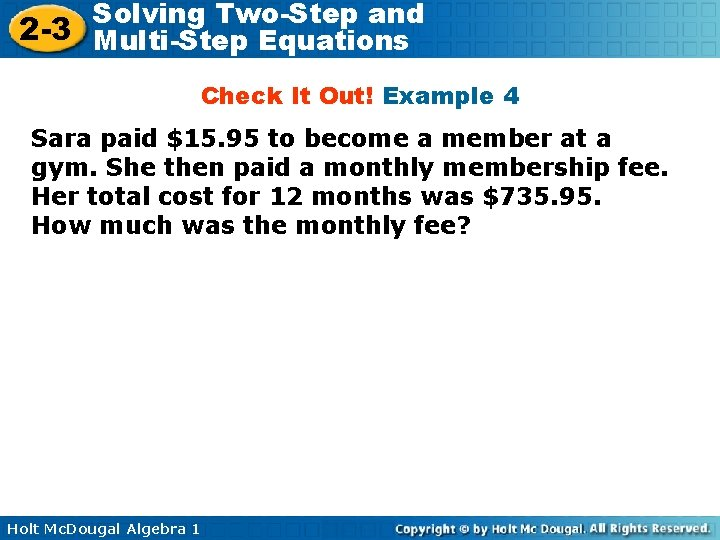Solving Two-Step and 2 -3 Multi-Step Equations Check It Out! Example 4 Sara paid \$15. 95 to become a member at a gym. She then paid a monthly membership fee. Her total cost for 12 months was \$735. 95. How much was the monthly fee? Holt Mc. Dougal Algebra 1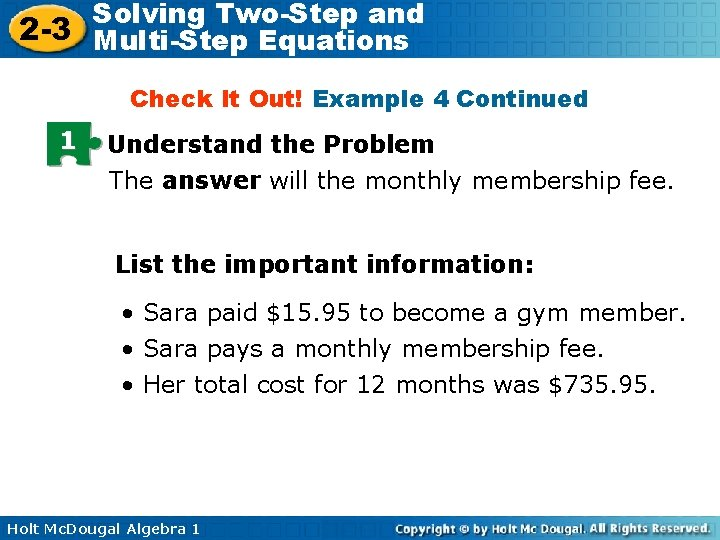Solving Two-Step and 2 -3 Multi-Step Equations Check It Out! Example 4 Continued 1 Understand the Problem The answer will the monthly membership fee. List the important information: • Sara paid \$15. 95 to become a gym member. • Sara pays a monthly membership fee. • Her total cost for 12 months was \$735. 95. Holt Mc. Dougal Algebra 1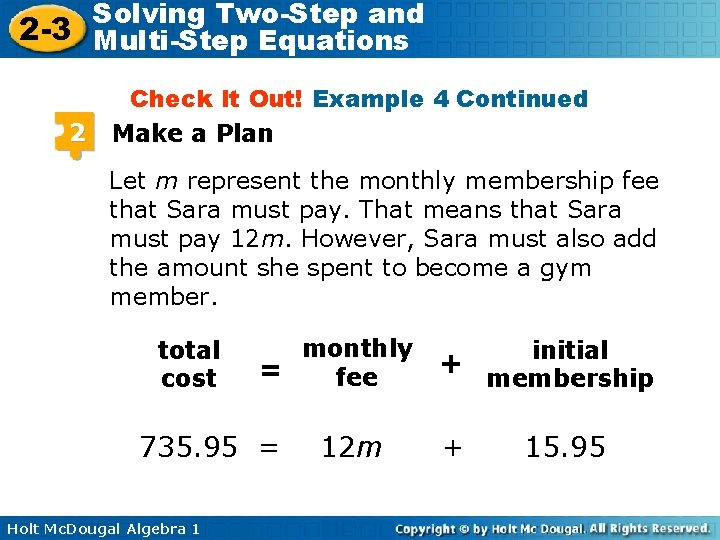Solving Two-Step and 2 -3 Multi-Step Equations 2 Check It Out! Example 4 Continued Make a Plan Let m represent the monthly membership fee that Sara must pay. That means that Sara must pay 12 m. However, Sara must also add the amount she spent to become a gym member. total cost monthly = fee 735. 95 = Holt Mc. Dougal Algebra 1 12 m initial + membership + 15. 95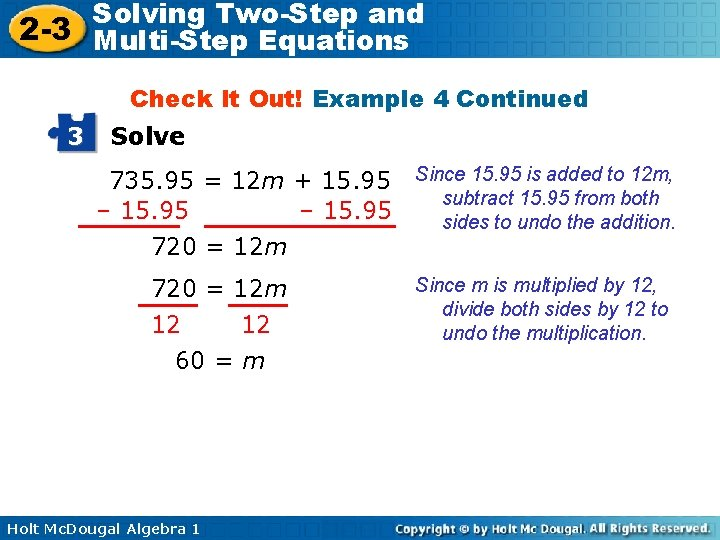Solving Two-Step and 2 -3 Multi-Step Equations Check It Out! Example 4 Continued 3 Solve 735. 95 = 12 m + 15. 95 Since 15. 95 is added to 12 m, subtract 15. 95 from both – 15. 95 sides to undo the addition. 720 = 12 m 12 12 60 = m Holt Mc. Dougal Algebra 1 Since m is multiplied by 12, divide both sides by 12 to undo the multiplication.Solving Two-Step and 2 -3 Multi-Step Equations Check It Out! Example 4 Continued 4 Look Back Check that the answer is reasonable. Sara pays \$60 a month, so after 12 months Sara has paid 12(60) = 720. Add the cost of the initial membership fee, which is about \$16. So the total paid is about \$736, which is close to the amount given in the problem, \$735. 95. Holt Mc. Dougal Algebra 1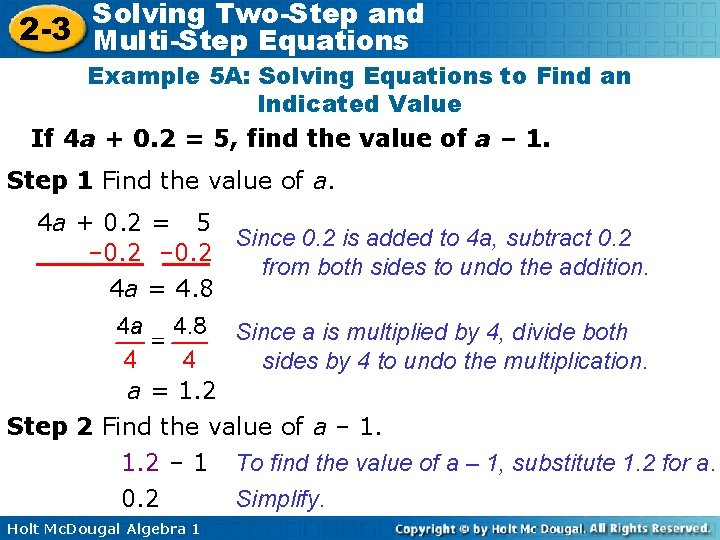Solving Two-Step and 2 -3 Multi-Step Equations Example 5 A: Solving Equations to Find an Indicated Value If 4 a + 0. 2 = 5, find the value of a – 1. Step 1 Find the value of a. 4 a + 0. 2 = 5 Since 0. 2 is added to 4 a, subtract 0. 2 – 0. 2 from both sides to undo the addition. 4 a = 4. 8 Since a is multiplied by 4, divide both sides by 4 to undo the multiplication. a = 1. 2 Step 2 Find the value of a – 1. 1. 2 – 1 To find the value of a – 1, substitute 1. 2 for a. Simplify. 0. 2 Holt Mc. Dougal Algebra 1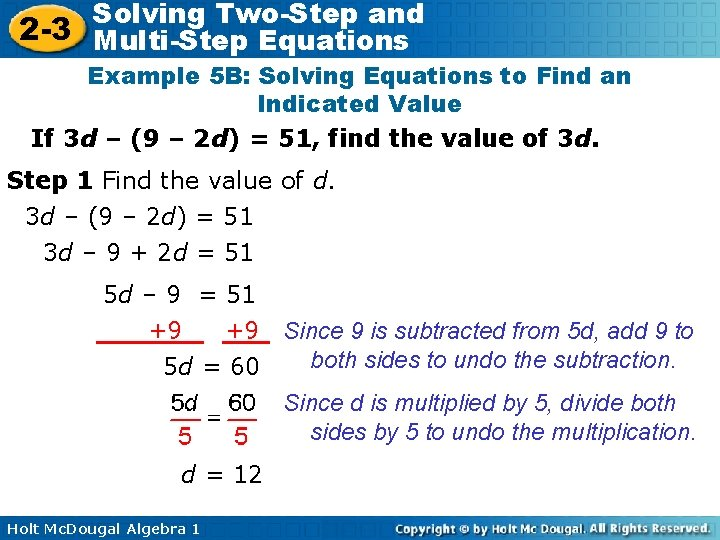Solving Two-Step and 2 -3 Multi-Step Equations Example 5 B: Solving Equations to Find an Indicated Value If 3 d – (9 – 2 d) = 51, find the value of 3 d. Step 1 Find the value of d. 3 d – (9 – 2 d) = 51 3 d – 9 + 2 d = 51 5 d – 9 = 51 +9 +9 Since 9 is subtracted from 5 d, add 9 to both sides to undo the subtraction. 5 d = 60 Since d is multiplied by 5, divide both sides by 5 to undo the multiplication. d = 12 Holt Mc. Dougal Algebra 1Solving Two-Step and 2 -3 Multi-Step Equations Example 5 B Continued If 3 d – (9 – 2 d) = 51, find the value of 3 d. Step 2 Find the value of 3 d. d = 12 3(12) 36 Holt Mc. Dougal Algebra 1 To find the value of 3 d, substitute 12 for d. Simplify.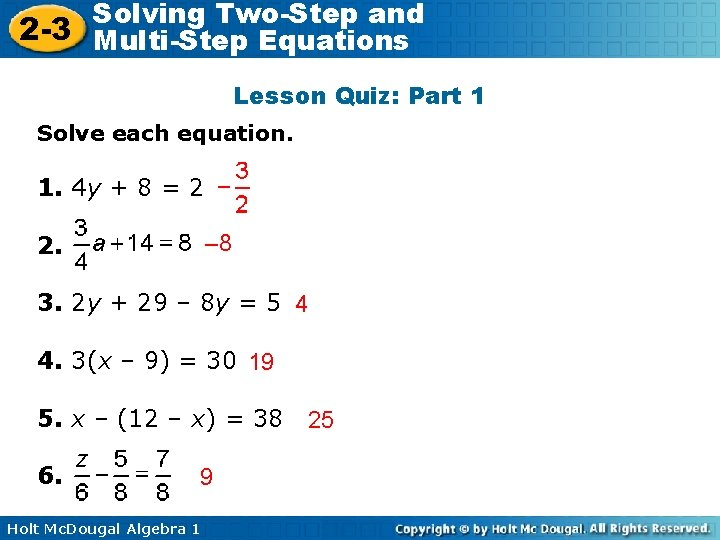Solving Two-Step and 2 -3 Multi-Step Equations Lesson Quiz: Part 1 Solve each equation. 1. 4 y + 8 = 2 – 8 2. 3. 2 y + 29 – 8 y = 5 4 4. 3(x – 9) = 30 19 5. x – (12 – x) = 38 6. 9 Holt Mc. Dougal Algebra 1 25Solving Two-Step and 2 -3 Multi-Step Equations Lesson Quiz: Part 2 7. If 3 b – (6 – b) = – 22, find the value of 7 b. – 28 8. Josie bought 4 cases of sports drinks for an upcoming meet. After talking to her coach, she bought 3 more cases and spent an additional \$6. 95 on other items. Her receipts totaled \$74. 15. Write and solve an equation to find how much each case of sports drinks cost. 4 c + 3 c + 6. 95 = 74. 15; \$9. 60 Holt Mc. Dougal Algebra 1